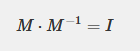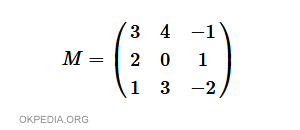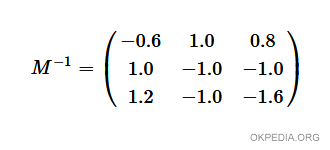# How to calculate the inverse matrix in Matlab and Octave

To calculate the inverse matrix in Matlab / Octave use the inv() function.

inv(m)

The parameter m is an invertible matrix.

The function returns the inverse matrix.

What is inverse matrix? The inverse M-1 matrix of a square matrix M is a matrix such that the product M-1 M is equal to the identity matrix I## Example

Given the following square matrix 3x3Define the matrix in the Matlab/Octave variable X

>> X = [3 4 -1; 2 0 1; 1 3 -2]

To calculate the inverse matrix use the inv() function.

>> Y=inv(X)

The function calculates and outputs the inverse matrix of X.The result is assigned to the variable Y.

-0.60000 1.00000 0.80000
1.00000 -1.00000 -1.00000
1.20000 -1.00000 -1.60000

Each element is a real number.

https://how.okpedia.org/en/matlab/how-to-calculate-the-inverse-matrix-in-matlab-and-octaveReport us an error or send a suggestion to improve this page

Matrix in Matlab/Octave